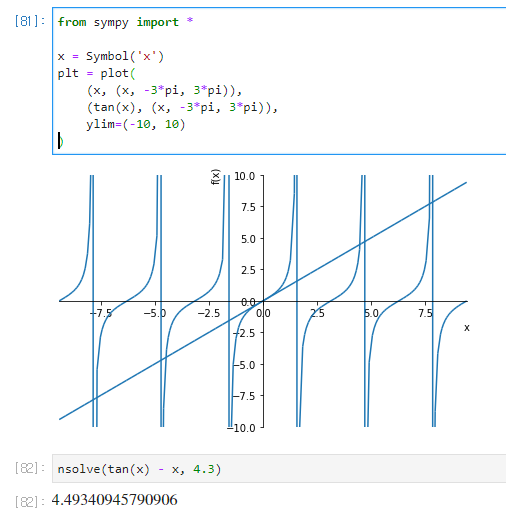# Problem with solve in handling some trigonometric equations #17162

Open
opened this issue Jul 7, 2019 · 3 comments

Projects
None yet
Contributor

### ishanaj commented Jul 7, 2019

 Recently, while using `solve()` to get the solution of some equations, I faced some problems with `solve`. I am not sure whether these are proper issues but I am not getting the desired result. ``````>>> solve(a*sin(x) - b*cos(x), x) [-2*atan((a - sqrt(a**2 + b**2))/b), -2*atan((a + sqrt(a**2 + b**2))/b)] `````` I think this should simply return `atan(b/a)`. Is there a way I could convert the answer in this form? Another problem: ``````>>> solve(tan(x) - x, x) File "C:\Python35\lib\site-packages\sympy\solvers\solvers.py", line 1162, in solve solution = _solve(f, *symbols, **flags) File "C:\Python35\lib\site-packages\sympy\solvers\solvers.py", line 1735, in _solve raise NotImplementedError('\n'.join([msg, not_impl_msg % f])) NotImplementedError: multiple generators [x, tan(x)] No algorithms are implemented to solve equation -x + tan(x) `````` Should it be giving an approximate solution like wolfram does here?
Contributor

### ethankward commented Jul 7, 2019

 `solve` does not give approximate solutions. You can `nsolve` though: ``````>>> nsolve(tan(x) - x, x, 1) 0.000348227174421857 ``````
Contributor Author

### ishanaj commented Jul 8, 2019

 Great! @ethankward Just another question. Since there would be many values that satisfy the equation `tan(x) - x`, so which value does `nsolve` return? I have referred the docs but I am still confused about it.
Member

### sylee957 commented Jul 9, 2019

 Most of the numerical root finding method, for example, newton's method, would need a certain initial guess to work, so you would have to see the plot manually and give a good seed to find a solution. I'm unsure about the whether the fully automated method to compute all the possible solutions, for equations like this, exists.However, I'm still confused about the reliability of nsolve, since it fails for some guesses like `nsolve(tan(x) - x, 4.1)` ``````--------------------------------------------------------------------------- ValueError Traceback (most recent call last) in ----> 1 nsolve(tan(x) - x, 4.1) ~\Documents\GitHub\sympy\sympy\utilities\decorator.py in func_wrapper(*args, **kwargs) 90 dps = mpmath.mp.dps 91 try: ---> 92 return func(*args, **kwargs) 93 finally: 94 mpmath.mp.dps = dps ~\Documents\GitHub\sympy\sympy\solvers\solvers.py in nsolve(*args, **kwargs) 3003 3004 f = lambdify(fargs, f, modules) -> 3005 x = sympify(findroot(f, x0, **kwargs)) 3006 if as_dict: 3007 return [{fargs: x}] ~\Miniconda3\envs\sympy_dev_37\lib\site-packages\mpmath\calculus\optimization.py in findroot(ctx, f, x0, solver, tol, verbose, verify, **kwargs) 977 '(%s > %s)\n' 978 'Try another starting point or tweak arguments.' --> 979 % (norm(f(*xl))**2, tol)) 980 return x 981 finally: ValueError: Could not find root within given tolerance. (1.09267558590813815048e-14 > 2.16840434497100886801e-19) Try another starting point or tweak arguments. ``````